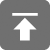# 工学1号馆

home

## Project Euler 5–Smallest multiple

Wu Yudong    September 26, 2017     欧拉计划   461

2520 is the smallest number that can be divided by each of the numbers from 1 to 10 without any remainder.

What is the smallest positive number that is evenly divisible by all of the numbers from 1 to 20?

2520是最小的能被1-10中每个数字整除的正整数。

#include <stdio.h>
#include <string.h>
#include <ctype.h>
#include <math.h>

#define N 20

int gcd(int a, int b)
{
if (b == 0)
return a;
else
return gcd(b, a % b);
}

int lcm(int a, int b)
{
return a / (gcd(a, b)) * b;
}

void solve()
{
int i, s = 2;
for (i = 3; i <= N; i++) {
s = lcm(s, i);
}
printf("%d\n", s);
}

int main()
{
solve();
return 0;
}